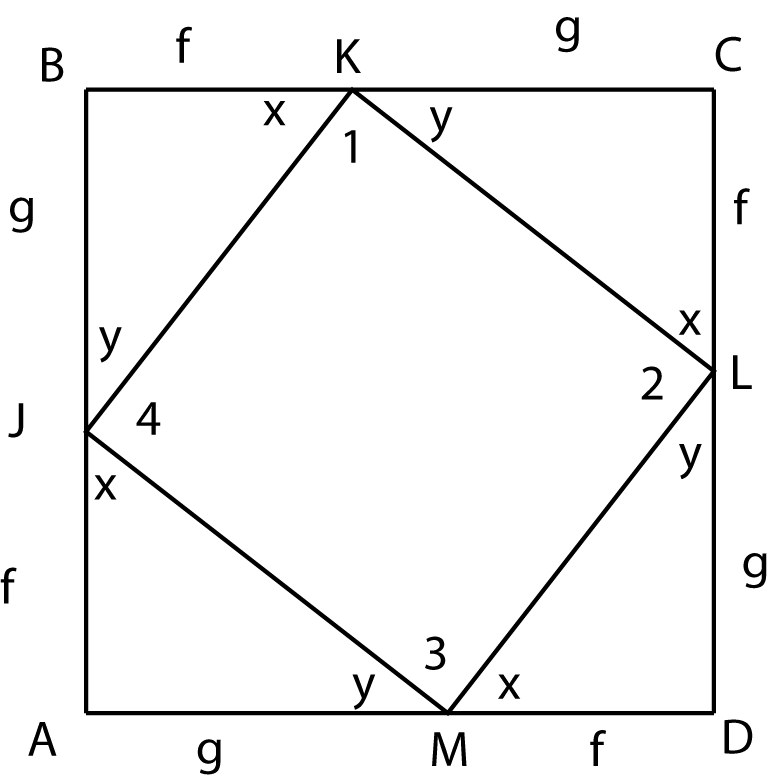### Prove: f^2 + g^2 = h^2Area postulate
Algebra
The triangle angle sum theorem
Algebra
Area of a square
Algebra
Definition
Algebra
SAS (Side-Angle-Side)
CPCTC
Algebra
Algebra
Definition
<B = <D = <C = <A = 90
Triangle JBK = Triangle KCL = Triangle LDM = Triangle MAJ
KJ = LK = ML = JM (label them h)
<X + <Y + <B = 180
<X + <Y = 90
<X + <Y + <1 = 180
<1 = 90 (repeat for angles 2, 3 and 4)
KLMJ is a square
Area of ADCB = (f + g)^2
Area of ADCB = area of the four triangles + area of KLMJ
Area of ADCB = 2fg + h^2
(f+g)^2 = 2fg + h^2
f^2 + 2fg + g^2 = 2fg + h^2
f^2 + g^2 = h^2